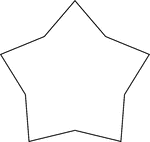### Concave Equilateral Decagon

A 5-point star made from a non-regular concave decagon in which all sides are equal in length.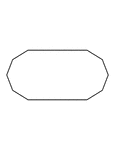### Irregular Convex Decagon

Illustration of an irregular convex decagon. This polygon has some symmetry.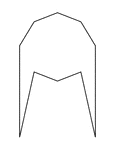### Irregular Convex Decagon

Illustration of an irregular convex decagon. This polygon has some symmetry.### Irregular Convex Decagon

Illustration of an irregular convex decagon. This polygon has some symmetry.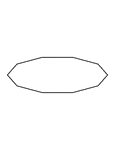### Irregular Convex Decagon

Illustration of an irregular convex decagon. This polygon has some symmetry.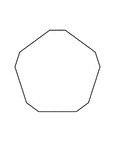### Irregular Convex Decagon

Illustration of an irregular convex decagon. This polygon has some symmetry.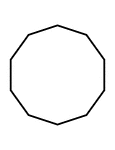### Flashcard of a Polygon with ten equal sides

A flashcard featuring an illustration of a Polygon with ten equal sides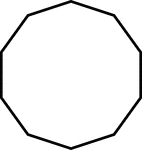### 10-sided Polygon

Polygon consisting of 10 sides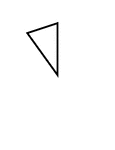### Fractions of 10-sided Polygon

1/10 of a 10 sided polygon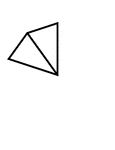### Fractions of 10-sided Polygon

2/10 of a 10 sided polygon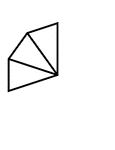### Fractions of 10-sided Polygon

3/10 of a 10 sided polygon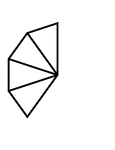### Fractions of 10-sided Polygon

4/10 of a 10 sided polygon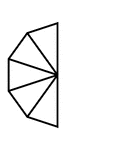### Fractions of 10-sided Polygon

5/10 of a 10 sided polygon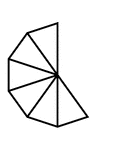### Fractions of 10-sided Polygon

6/10 of a 10 sided polygon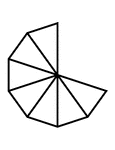### Fractions of 10-sided Polygon

7/10 of a 10 sided polygon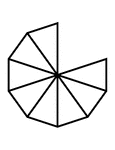### Fractions of 10-sided Polygon

8/10 of a 10 sided polygon### Fractions of 10-sided Polygon

9/10 of a 10 sided polygon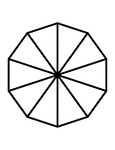### Fractions of 10-sided Polygon

10/10 of a 10 sided polygon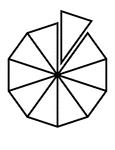### Fractions of 10-sided Polygon

10/10 of a 10 sided polygon with one piece detached